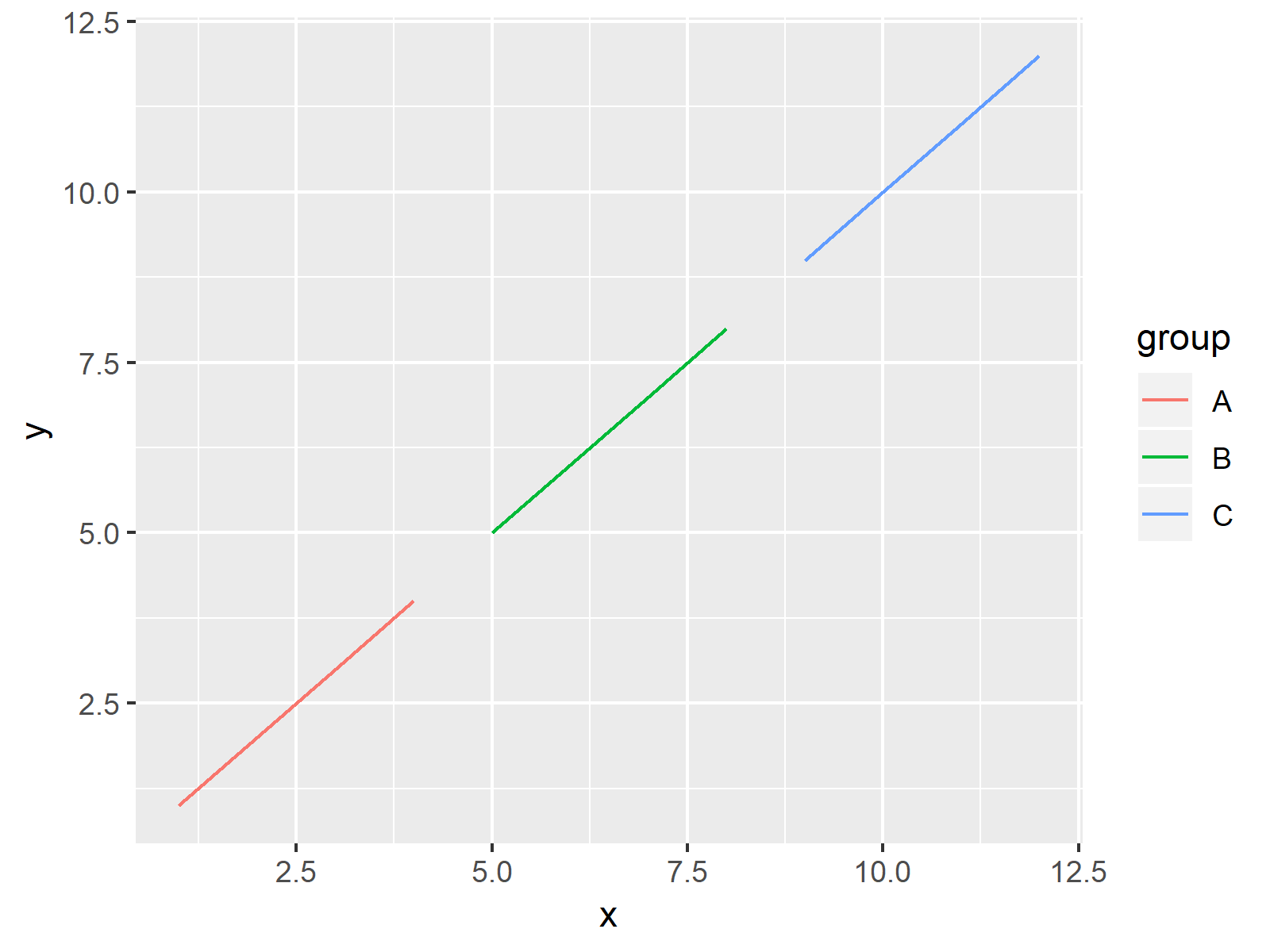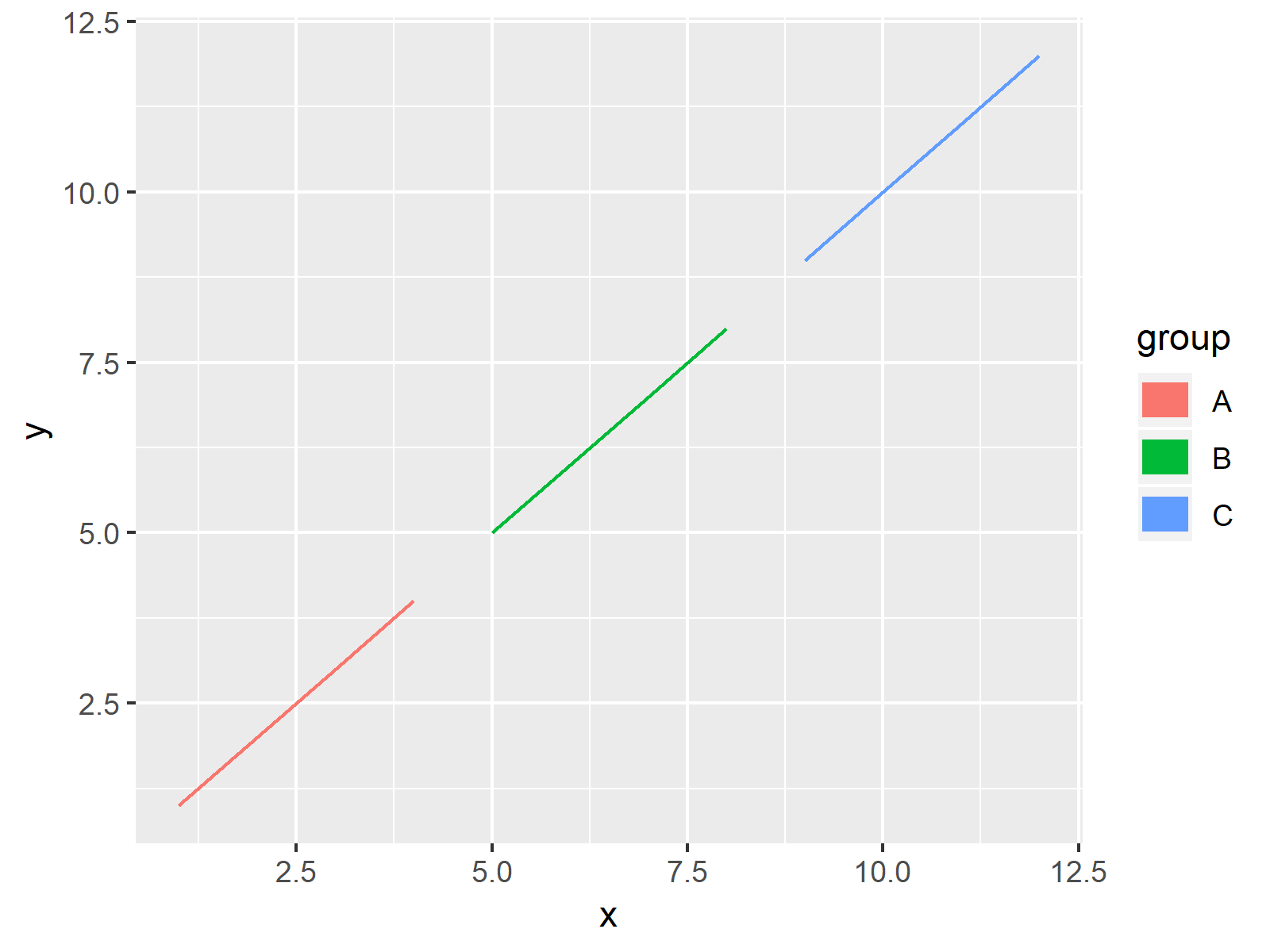# Control Size of ggplot2 Legend Items in R (Example) | How to Adjust Symbols

This tutorial explains how to adjust the size of ggplot2 legend symbols in R.

The tutorial contains this:

Here’s how to do it!

## Example Data, Add-On Packages & Default Plot

Have a look at the following example data:

```data <- data.frame(x = 1:12,                     # Example data
y = 1:12,
group = c(rep("A", 4),
rep("B", 4),
rep("C", 4)))
data                                             # Print data
#     x  y group
# 1   1  1     A
# 2   2  2     A
# 3   3  3     A
# 4   4  4     A
# 5   5  5     B
# 6   6  6     B
# 7   7  7     B
# 8   8  8     B
# 9   9  9     C
# 10 10 10     C
# 11 11 11     C
# 12 12 12     C```

The previous output of the RStudio console shows that our example data has 12 rows and 3 columns.

In this tutorial, we’ll also need to install and load the ggplot2 package:

```install.packages("ggplot2")                      # Install & load ggplot2
library("ggplot2")```

Now, we can draw our data as follows:

```ggp <- ggplot(data, aes(x, y, col = group)) +    # Basic ggplot2 plot
geom_line()
ggp                                              # Draw plot```The output of the previous R syntax is shown in Figure 1: A basic ggplot2 line plot with default size of legend items.

## Example: Change Size of Legend Items Using guides Function

This Example shows how to control, the size of our legend items without changing the plot itself. For this task, we can use the guides and the guide_legend functions provided by the ggplot2 package:

```ggp +                                            # Apply guides function
guides(color = guide_legend(override.aes = list(size = 5)))```As shown in Figure 2, the previous syntax created a ggplot2 graphic with user-defined sizes of legend items.

## Video & Further Resources

Do you need more info on the R syntax of this tutorial? Then you may watch the following video of my YouTube channel. In the video, I’m explaining the contents of this article in a programming session:

Please accept YouTube cookies to play this video. By accepting you will be accessing content from YouTube, a service provided by an external third party.If you accept this notice, your choice will be saved and the page will refresh.

In addition, you may read some of the other articles on statisticsglobe.com. You can find a selection of articles below.

In this tutorial, I showed how to modify the legend item size in a ggplot2 graph in R.In the present tutorial, we have used a line plot to illustrate how to increase or decrease the size of legend items. However, we may use a similar syntax to adjust the item size of other legend items such as points and boxes as well. Let me know in the comments, in case you have further questions.

Subscribe to the Statistics Globe Newsletter

•Joel
April 26, 2023 9:52 pm

Excellent, this is the way to go.

•April 27, 2023 8:09 am